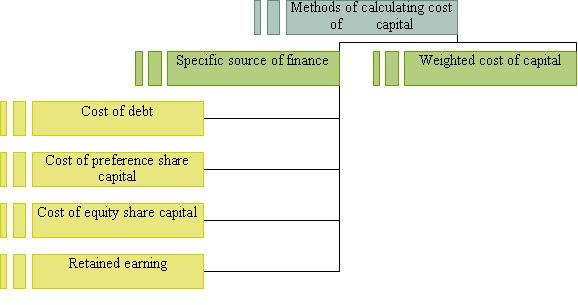# Computation or methods of calculating cost of capital

The cost of capital can be calculated by different methods these are discussed as below:

(I) Computation of cost by specific source of finance

1) Cost of debt: cost of debt means the interest payable on the debentures. For example, if the company issue Rs 40000, 10% debentures at par in that case before tax cost of debt will be

Kdb=I/P

=4000/40000

=1/10

If the debt is raised at discount or premium than the P (principal) will be changed to NP   (Net proceeds).

Kdb=I/NP

And in case the firm has made payment of taxes than it will also be deducted.

Kdb=I/NP (1-t)

And if there are redeemable debentures than

Kdb= I+1/n(RV-NP)/1/2(RV-NP)

2.) Cost of preference share capital: a fixed rate of dividend is paid to the preference shareholders at regular intervals. If it is not paid to them than it affects the fund raising capacity of the firm.

Kp =D/P

If the preference shares has been issued at discount or premium than the P (principal) will be changed to NP (Net proceeds).

Kp=D/NP

And if there are redeemable preference shares than

Kp= D+1/n(MV-NP)/1/2(MV-NP)

3.) Cost of equity share capital: it is not legally binding upon the company to pay dividend to the equity shareholders. They are not paid dividend at fixed rate. Shareholders invest money with the hope that they will get the dividend and the firm must earn minimum rate of return to keep the market price of shares stable. The return may be in different form

a) dividend per share

Ke =D/P

b) Dividend per share + growth

Ke =D/P + g

c) Earning per share

Ke =EPS/NP

d) Realized yield method: the earlier methods fail to estimate future dividend and earning correctly. To overcome this limitation actual average rate of return realized in the past is used. Average rate of return is found out with the help of dividend received in the past along with gain realized at the time of the sale of the shares.

4.) Cost of retained earning: it is the opportunity cost of dividend foregone by the shareholders.

Kr =D/NP + g

(II) Weighted average cost of capital: it is also called overall cost of capital or composite cost of capital as it is the composite cost of various source of capital. In this kind of cost of capital weights are given to specific cost of capital. The weights may have book value or market value. As market value represents the true value of the investors, so market value weights are preferred than the book value.

Kw =∑ XW/∑W